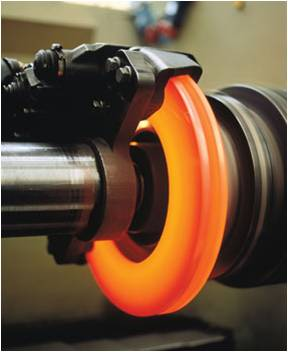# Braking

(Redirected from Brake)The thermal energy generated during friction braking can be seen in this friction brake/rotor set-up during stress testing.

Braking is the process of controlling the velocity of an object by inhibiting its motion. An object in motion possesses kinetic energy and to bring the object to a stop this kinetic energy must be removed. Removing the kinetic energy can be accomplished by dissipating the energy to the atmosphere through friction or by converting it into another form of energy. The most common type of braking is a mechanical brake which inhibits motion through friction brake pads. A mechanical brake applies a friction force to convert the kinetic energy of the vehicle into thermal energy which then dissipates into the atmosphere. Braking systems which don't use friction are referred to as regenerative braking systems (RBSs). In RBSs, the kinetic energy is converted into other forms of useful energy, which can be stored for later use, increasing fuel efficiency.

## Background

As with any system, the process of braking must follow the principal of conservation of energy. Energy cannot be created or destroyed but only converted from one form to another,

The energy present in an object in motion is given by the following equation:

where,

is the mass of the object in kilograms (kg).

is the velocity of the object in meters per second (m/s).

is the kinetic energy in joules (J)

From this equation, and assuming the mass of the object is constant, it is clear the in order to remove the kinetic energy from the system, the velocity must be brought to zero.

## Friction braking

Friction braking is the most commonly used braking method in modern vehicles. It involves the conversion of kinetic energy to thermal energy by applying friction to the moving parts of a system. The friction force resists motion and in turn generates heat, eventually bringing the velocity to zero. The energy taken from the system is given by the following equation:

where,

is the force of friction in newtons (N).

is the stopping distance in meters (m).

is the thermal energy produced by the brakes in Joules.

Applying conservation of energy to the above two equations, the thermal energy produced must equal the kinetic energy dissipated:

;

;

From this equation it can be seen that increasing the velocity or mass of an object means the applied friction force must be increased to bring the object to a stop in the same distance.

## Braking in motor vehicles

### Mechanical braking

The most common method of braking in motor vehicles is mechanical or friction braking. In this method, some or all of the vehicles wheels are fitted with brake pads which apply a friction force that inhibits the motion of the wheels. Frictional braking results in a conversion of the kinetic energy gained from fuel consumption to thermal energy. This thermal energy then dissipates to the atmosphere in the form of waste heat.

Large friction forces can be needed to inhibit a vehicle's motion, particularly in large machines and trucks which have a high mass and therefore high kinetic energy. The brake pads, which are responsible for applying the friction force, experience wear over the course of their life due to this friction force. The brake pad wear causes the brakes to become less effective over the course of their life and need regular replacement.

### Regenerative braking

main article

Regenerative braking system involves the removal of the kinetic energy of a moving object by converting it into another form of useful energy, such as electric, pneumatic, or stored kinetic energy. The use of regenerative braking can increase the overall efficiency of a motor vehicle by conserving some of its kinetic energy which can then be used to bring the vehicle back up to speed.